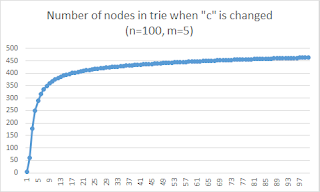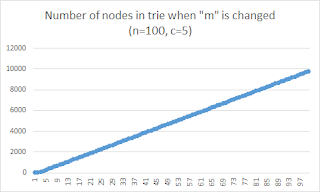## Friday, August 7, 2015

### Predicting the number of nodes in a trie with uniformly distributed strings

A trie is a type of tree that stores strings. Each character of the strings is a node and strings that share a common prefix also share the nodes, which means that a common prefix is only stored once, reducing some redundancy. But how much space is saved by using a trie? In order to answer this question, first we have to calculate the expected number of nodes a trie will have for "n" strings of "m" characters each with "c" possible characters (character set).

Consider the following diagram of a trie that contains the words "me", "if", "in", and "it". In it we have added a new word "my".The word "my" only required the creation of one new node, since its first letter already existed in the word "me" so that node was shared and not recreated. In general, if a string is inserted in a trie, the number of new nodes created depends on the length of the longest existing prefix in the trie. This length will be the number of nodes that will be shared/reused. The remainder of the string will require new nodes for each character. If the whole string already exists then there will be 0 new nodes whilst if the string is completely new with no existing prefix then there will be a new node for each character. Specifically, for a string of length "m" whose longest existing prefix is of length "p", the number of new nodes created will be "m - p".

The equation we need to figure out looks like the following:
expected number of nodes = (m)(expected number of strings with prefix of length 1 not found)
+ (m-1)(expected number of strings with prefix of length 2 not found)
+ (m-2)(expected number of strings with prefix of length 3 not found)
+ ...
+ (1)(expected number of strings with prefix of length m not found)

Assuming that the strings are generated using a uniform distribution (any character can appear anywhere in the string), we need to find the expected number of strings out of "n" inserted strings made from "c" possible characters that will have a non-existing prefix of length "p".

This is basically the expected number of strings being selected for the first time when "n" selections are made from among all possible "p" length strings made from "c" possible characters (there are "c^p" possible such prefixes). This is equivalent to saying that it is the expected number of non-collisions when randomly placing "n" objects in "c^p" slots.

In my previous post, I showed that the expected number of collisions when randomly placing "n" objects in "s" slots is
n - s(1 - (1 - 1/s)^n)
which means that the number of non-collisions is
n - (n - s(1 - (1 - 1/s)^n))
which simplifies to
s(1 - (1 - 1/s)^n)
which when we plug in our values becomes
(c^p)(1 - (1 - 1/(c^p))^n)

But there's a problem. The above equation tells you the expected number of non-collisions when considering "p" length prefixes. But consider the previous diagram again. If the word "he" was added, it is true that the length 2 prefix of the word ("he") does not result in a collision, but this does not mean that just 1 new node will be added. In reality, 2 new nodes will be added because it is also true that its length 1 prefix ("h") will also not result in a collision. What this means is that the equation will not give the number of strings which will not result in a collision due to their length "p" prefix only, but also due to their length "p-1" prefix, which is not what we want. To fix this, we subtract from the equation the number of non-collisions due to the shorter prefix:
expected number of strings with prefix of length p not found = (c^p)(1 - (1 - 1/(c^p))^n) - (c^(p-1))(1 - (1 - 1/(c^(p-1)))^n)

Of course this does not apply for the length 1 prefix, so we need to be careful to only apply the subtraction for prefix lengths greater than one.
(You might think that we need to subtract for each shorter prefix length, but when this was tried the result became a negative number. Perhaps some form of inclusion-exclusion principle needs to be applied. Using this equation, the result matches empirical data for many different parameters.)

So, continuing from our earlier equation,
expected number of nodes = (m)((c^1)(1 - (1 - 1/(c^1))^n))
+ (m-1)((c^2)(1 - (1 - 1/(c^2))^n) - (c^(2-1))(1 - (1 - 1/(c^(2-1)))^n))
+ (m-2)((c^3)(1 - (1 - 1/(c^3))^n) - (c^(3-1))(1 - (1 - 1/(c^(3-1)))^n))
+ ...
+ (1)((c^m)(1 - (1 - 1/(c^m))^n) - (c^(m-1))(1 - (1 - 1/(c^(m-1)))^n))
= sum( c^i - c^i*((c^i-1)/c^i)^n for i in 1..m )


In Python code this becomes:
from fractions import Fraction
def exp_num_trie_nodes(n,m,c):
return float(sum( c**i - c**i*Fraction(c**i-1,c**i)**n for i in range(1,m+1) ))

Rate of change

Here is a comparison of how the number of nodes increases depending of which variable (n,m,c) is changed:As "n" increases, the number of nodes added starts slowing down, which makes sense since the more strings there are, the more existing prefixes can be reused. As "m" increases, the number of nodes added starts speeding up and then becomes linear, which makes sense too since longer strings are sparser and thus it would be harder to find a matching prefix which is long from among 100 strings. As "c" increases, the number of nodes added shoots up until a point where it then slows down, almost like it is logarithmic. This is because after a point it will not matter how rare the strings are since there are only 100 strings to choose from among the "c^m" possible strings. Since the length is not increasing, the same number of nodes will be used.

Size of trie

So does using a trie compress a set of strings? Keep in mind that a node takes more space than a character since it needs to point to other nodes whereas strings are arrays without pointers. We'll assume that all strings are the same length in order to reduce the number of variables. This will reduce the amount of information needed for both the set of strings and the trie (no need to include terminator flags for the strings) and the number of strings of maximum length is greater than the total number of shorter strings so it will not be a significant error in representation.

Call the number of nodes in the trie "N(n,m,c)".

The size of the normal set of strings is as follows:
n(m log(c))
where "log(c)" is the size of each character (the number of bits needed to represent each character). Of course this assumes that each string is unique. Tries only store unique strings and the way we compute the number of nodes does not assume that the strings will be unique. So we need to subtract the expected number of repeated strings from among those "n" strings. The number of repeated strings is equal to the number of collisions when placing "n" objects in "c^m" slots.
Array of strings: n(m log(c)) - (n - (c^m)(1 - (1 - 1/(c^m))^n))

The size of the trie is as follows:
N(n,m,c)(k(log(c) + log(N(n,m,c))))
where "log(c)" is the size of each character (the number of bits needed to represent each character), "log(N(n,m,c))" is the size of a pointer (which at minimum would be the logarithm of the number of nodes), and "k" is the number of pointers used on average per node. Given that the majority of the nodes in a trie will be leaf nodes, the majority of nodes will not have children. In fact the average will be less than one child per node. If arrays are used, "k" must be equal to "c", but if a linked list is used then "k" is the average but we have to also include the linked list pointer size with each character. The pointer size of the linked lists can be assumed to be "log(N(n,m,c))" since the total number of child nodes is equal to the number of nodes (minus the root node).
Array based: N(n,m,c)(c(log(c) + log(N(n,m,c))))
Linked list based: N(n,m,c)(k(log(c) + log(N(n,m,c)) + log(N(n,m,c))))

Here is a graph showing how the set of strings, array based trie, and linked list based trie increase in size with "n" when "c" is 5, "m" is 5, and "k" is 0.9:It is clear that an array based trie cannot be used to compress a collection of strings as nodes take too much space. But what if we changed the value of "k" in the linked list based trie?This shows that unless you have an average number of children per node of 0.2 or less, the array of strings will always take less space. Notice that this says nothing about tries which attempt to minimize the number of nodes such as radix trees where a single node represents a substring rather than a character. Also notice that this is about uniformly distributed strings, not linguistic strings which have a lot of redundancy. In a future post I shall make empirical comparisons on linguistic data.